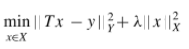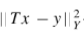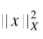# Tikhonov Regularization: Simple Definition

Share on

## What is Tikhonov Regularization?

Tikhonov Regularization (sometimes called Tikhonov-Phillips regularization) is a popular way to deal with linear discrete ill-posed problems. Ill-posed problems violate one of the terms of a well-posed problem. Well posed problems:

• Have a solution.
• Have a solution that is unique.
• Have a solution that changes as the initial condition changes.

Regularization stabilizes ill­-posed problems, giving accurate approximate solutions — often by including prior information (Vogel).

For example, let’s say you wanted to find a vector x so that Ax = b. You could use ordinary least squares to find a solution, but you may find that no solutions exist, or multiple solutions exist. Tikhonov Regularization can give you a meaningful, approximate solution to this ill-posed problem.

Tikhivov’s method can produce solutions even when the data set contains a lot of statistical noise. It is essentially the same technique as ridge regression; The main difference is that Tikhonov’s has a larger set.

## The Method

Tikhobv’s method uses the following problem in place of the problem that calculates the minimum-norm least squares:Where λ is the regularization parameter, which specifies the amount of regularization. λ essentially acts as a Lagrange multiplier, in that you are solving a minimization problem with ‖x‖ = R acting as a constraint for some R.
The basic idea is to make:as small as possible (without minimizing it), without:becoming too big.

More formally, the definition (from Kaipio) is:

Let λ > 0 be a given constant. The Tikhonov regularized
solution xλ ∈ H1 is(the minimizer of the given function) provided that a minimizer exists.

The proof is beyond the scope of this site, but you can find an excellent outline in JP Kaipo’s Classical Regularization Methods, available as a pdf here.

## Choosing λ

Choosing a value for λ can be challenging. Several methods exist for calculating a suitable values including L-curve methods and the Morozov discrepancy principle (discussed in Kaipo).

References:
Alessandro Buccini. Regularizing preconditioners by non-stationary iterated Tikhonov with general penalty term.
Mark Gockenbach. Linear Inverse Problems and Regularization.
Curt Vogel. Regularization Methods.
JP Kaipio. “Classical Regularization Methods.” Statistical and Computational Inverse Problems. Volume 160 of the series Applied Mathematical Sciences pp 7-48.

CITE THIS AS:
Stephanie Glen. "Tikhonov Regularization: Simple Definition" From StatisticsHowTo.com: Elementary Statistics for the rest of us! https://www.statisticshowto.com/tikhonov-regularization/
------------------------------------------------------------------------------

Need help with a homework or test question? With Chegg Study, you can get step-by-step solutions to your questions from an expert in the field. Your first 30 minutes with a Chegg tutor is free!